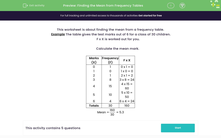# Finding the Mean from Frequency Tables

In this worksheet, students review how to find the mean from frequency tables.Key stage:  KS 3

Curriculum topic:   Statistics

Curriculum subtopic:   Construct/Interpret Appropriate Representations for Data

Difficulty level:#### Worksheet Overview

This worksheet is about finding the mean from a frequency table.

Example

The table gives the test marks out of 6 for a class of 30 children.

F x X is worked out for you.

Calculate the mean mark.

 Marks (X) Frequency (F) F x X 0 1 0 x 1 = 0 1 0 1 x 0 = 0 2 1 2 x 1 = 2 3 8 3 x 8 = 24 4 15 4 x 15 = 60 5 10 5 x 10 = 50 6 4 6 x 4 = 24 Totals 30 160
 Mean = 160 = 5.3 30

### What is EdPlace?

We're your National Curriculum aligned online education content provider helping each child succeed in English, maths and science from year 1 to GCSE. With an EdPlace account you’ll be able to track and measure progress, helping each child achieve their best. We build confidence and attainment by personalising each child’s learning at a level that suits them.

Get started